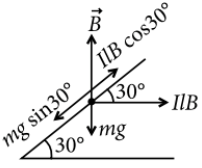Motional EMF
Question

# A metallic rod of mass per unit length 0.5 kg ${m}^{-1}$ is lying horizontally on a smooth inclined plane which makes an angle of ${30}^{0}$ with the horizontal. The rod is not allowed to slide down by flowing a current through it when a magnetic field of induction 0.25 T is acting on it in the vertical direction. The current flowing in the rod to keep it stationary is

Moderate
Solution

## Mass per unit length of a metallic rodLet I be the current flowing. For equilibrium,$mg\mathrm{sin}30°=IlB\mathrm{cos}30°$

Get Instant Solutions